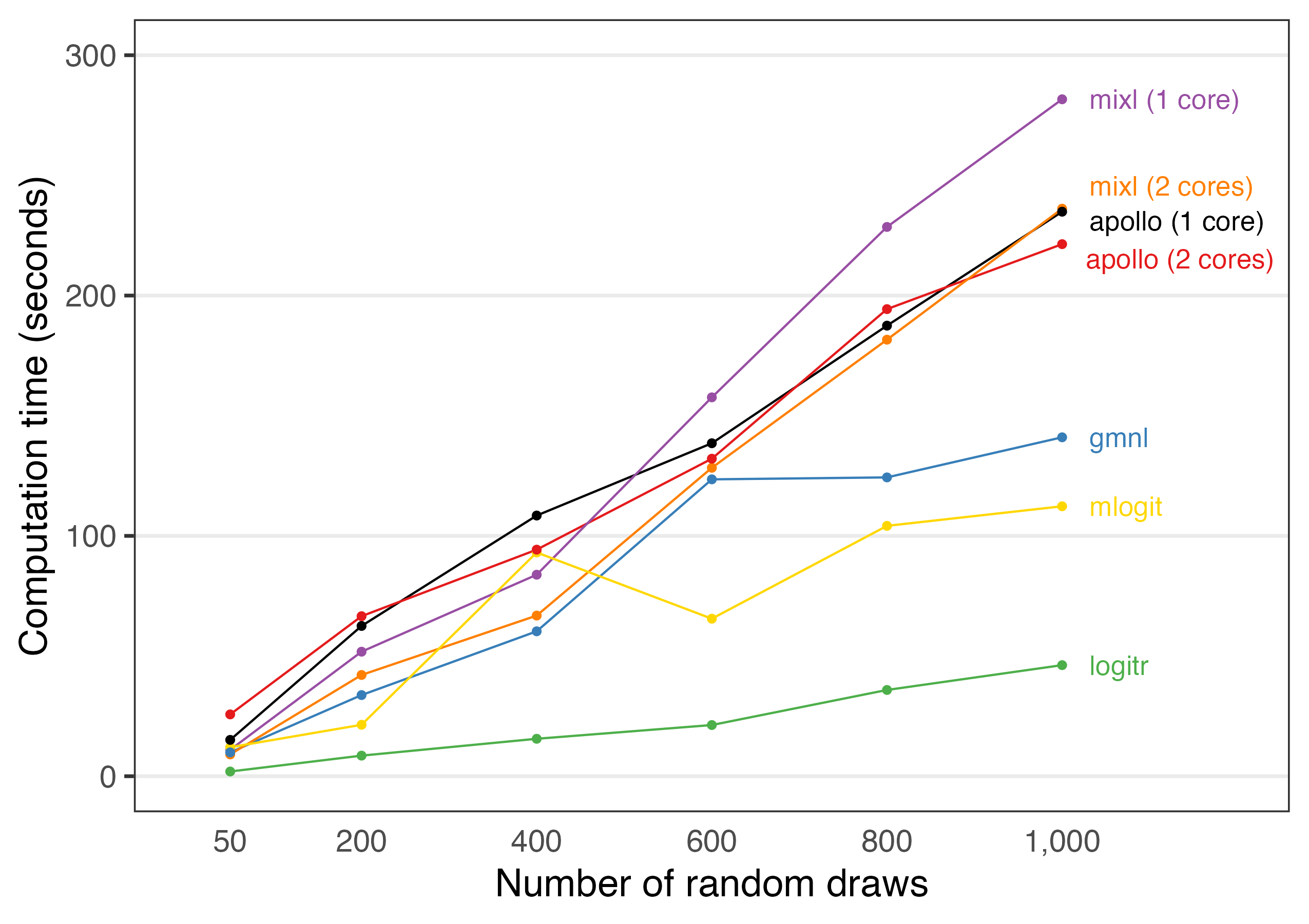{logitr} is faster than most other packages with similar functionality. To demonstrate this, a benchmark was conducted by estimating the same preference space mixed logit model using the following R packages:

• {logitr}
• {mixl}
• {mlogit}
• {gmnl}
• {apollo}

The benchmark can be viewed at this Google Colab notebook:

Benchmarks will always vary for every run of a benchmarking code, even when run on the same machine due to variations in background processes. Thus, if you run this code yourself on a different machine, your results may vary, though the overall order and trends in terms of each package’s relative speed should be similar to those from the Colab notebook.

## Comparing run times

The {logitr} package includes a runtimes data frame that is exported from the Google Colab notebook used to conduct the benchmark. The tables below summarize the run times for each package and how many times slower they are relative to {logitr}.

library(logitr)
library(dplyr)
library(tidyr)
library(kableExtra) # For tables

numDraws <- unique(runtimes\$numDraws)
logitr_time <- runtimes %>%
filter(package == "logitr") %>%
rename(time_logitr = time_sec)
time_compare <- runtimes %>%
left_join(select(logitr_time, -package), by = "numDraws") %>%
mutate(mult = round(time_sec/ time_logitr, 1)) %>%
select(-time_logitr)
# Compare raw times
time_compare %>%
select(-mult) %>%
pivot_wider(names_from = numDraws, values_from = time_sec) %>%
kbl()
package 50 200 400 600 800 1000
logitr 2.752860 8.930408 13.72697 24.13735 33.47056 39.22012
mixl (1 core) 10.640267 49.667703 80.22922 158.23673 229.17401 271.35824
mixl (2 cores) 8.928487 41.577738 66.05436 129.84954 184.54557 230.91617
mlogit 11.926502 19.901097 87.58429 60.38106 100.63138 98.41650
gmnl 10.553787 31.379011 69.74575 121.93843 99.23701 141.36727
apollo (1 core) 17.287605 44.118732 84.29395 129.33302 164.37400 198.21812
apollo (2 cores) 21.911355 53.223971 82.69647 120.27101 163.80518 196.84896
# Compare how many times slower compared to logitr
time_compare %>%
select(-time_sec) %>%
pivot_wider(names_from = numDraws, values_from = mult) %>%
kbl()
package 50 200 400 600 800 1000
logitr 1.0 1.0 1.0 1.0 1.0 1.0
mixl (1 core) 3.9 5.6 5.8 6.6 6.8 6.9
mixl (2 cores) 3.2 4.7 4.8 5.4 5.5 5.9
mlogit 4.3 2.2 6.4 2.5 3.0 2.5
gmnl 3.8 3.5 5.1 5.1 3.0 3.6
apollo (1 core) 6.3 4.9 6.1 5.4 4.9 5.1
apollo (2 cores) 8.0 6.0 6.0 5.0 4.9 5.0

The code below plots the relative run times from the Colab notebook.

library(ggplot2)
library(ggrepel)

plotColors <- c("black", RColorBrewer::brewer.pal(n = 5, name = "Set1"), "gold")
benchmark <- runtimes %>%
ggplot(aes(x = numDraws, y = time_sec, color = package)) +
geom_line() +
geom_point() +
geom_text_repel(
data = . %>% filter(numDraws == max(numDraws)),
aes(label = package),
hjust = 0, nudge_x = 40, direction = "y",
size = 4.5, segment.size = 0
) +
scale_x_continuous(
limits = c(0, 1200),
breaks = numDraws,
labels = scales::comma) +
scale_y_continuous(limits = c(0, 300), breaks = seq(0, 300, 100)) +
scale_color_manual(values = plotColors) +
guides(
point = guide_legend(override.aes = list(label = "")),
color = guide_legend(override.aes = list(label = ""))) +
theme_bw(base_size = 18) +
theme(
panel.grid.minor = element_blank(),
panel.grid.major.x = element_blank(),
legend.position = "none",
axis.line.x = element_blank(),
axis.ticks.x = element_blank()
) +
labs(
x = "Number of random draws",
y = "Computation time (seconds)"
)

benchmark